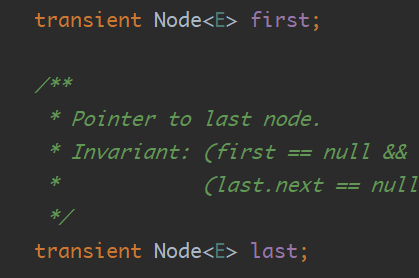## 题目描述

s = "3[a]2[bc]", 返回 "aaabcbc".
s = "3[a2[c]]", 返回 "accaccacc".
s = "2[abc]3[cd]ef", 返回 "abcabccdcdcdef".


## 解法

java

  //解析字符串，遇到数字进入栈，遇到[ 进入 遇到] 开始输出
public String decodeString(String s) {
char[] sArr= s.toCharArray();
int num = 0;
StringBuffer res=new StringBuffer();
for (char c : sArr) {
//获取到数字
if(Character.isDigit(c)){
num  = num*10+Integer.parseInt(String.valueOf(c));
} else if(c=='['){
//将获取到的数字存入到栈，并且存入之前的字符，开始重写计数
//stackNum和stackString stackNum[i] stackString[i]记录之前的字符串
num=0;
res =new StringBuffer();
}else if(c==']'){
StringBuffer temp=new StringBuffer();
int temp_num=stackNum.removeLast();
//cc
for (int i = 0; i < temp_num; i++) {
temp.append(res);
}
//合并到acc
res = new StringBuffer(stackString.removeLast() + temp);
}else if(Character.isLetter(c)){
res.append(c);
}

}
return res.toString();
}


C++

public:
static string decodeString(string s){
size_t num = 0;
vector<int> stackNum;
vector<string> stackString ;
string res;
for (int i = 0; i < s.size(); i++) {
char c=s[i];
if (isdigit(c)) {
//用char进行计算时，需要减去48.字符转换为数值
num=num*10+c - '0';
}else if(c=='['){
stackNum.push_back(num);
stackString.push_back(res);
num=0;
res ="";
}else if(c==']'){
int count = stackNum.back();
stackNum.pop_back();
string resTemp=res;
for (int k = 1; k < count; k++)
{
resTemp.append(res);
}
res = stackString.back() + resTemp;
stackString.pop_back();
}else if(isalpha(c)){
//是否为字母
res.push_back(c);
}
}
return res;
}


## 解题思路

### 栈

1.分析实例

3[a2[c]]=> 3[acc]=>accacc
1.1解析内部的2[c]

1.2再解析外部3[acc]

*3\r\n
$3\r\n foo\r\n$-1\r\n
\$3\r\n
bar\r\n


2.寻找规则

 s = "3[a]2[bc]", 返回 "aaabcbc".


s = "3[a2[c]]", 返回 "accaccacc"


2.1从左往右读取规则
2.2最先读取的规则，最后输出
2.3一个完整[]，表示一个输出字段，遇到[开始记录，到]结束。

3.代码实现
3.1 定义两个栈，一个记录数字，一个记录字符LinkedList<Integer> stackNum = new LinkedList<>();


 StringBuffer res=new StringBuffer();


3.1遍历读取字符

char[] sArr= s.toCharArray();
for (char c : sArr) {
}

##### 读取数字字节
if(Character.isDigit(c)){
num  = num*10+Integer.parseInt(String.valueOf(c));
}


//遇到[
else if(c=='['){
//遇到]
}else if(c==']'){
//遇到字符字节
}else if(Character.isLetter(c)){
}

##### 处理字符字节

###### 遇到[

num=0;
res =new StringBuffer();


 stackNum.add(num);

###### 遇到]

int temp_num=stackNum.removeLast();


StringBuffer temp=new StringBuffer();
for (int i = 0; i < temp_num; i++) {
temp.append(res);
}


res = new StringBuffer(stackString.removeLast() + temp);


###### 字节

else if(Character.isLetter(c)){
res.append(c);
}